# Principles-of-Thermodynamics

22. Sep 2022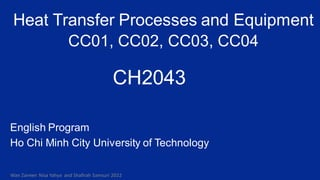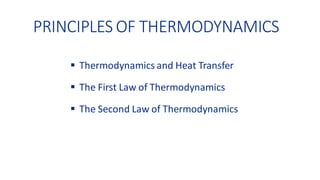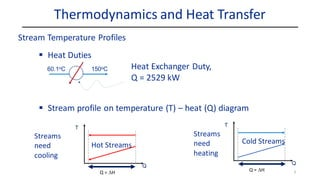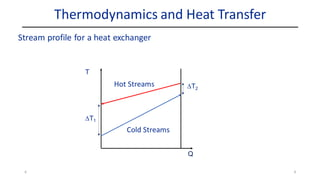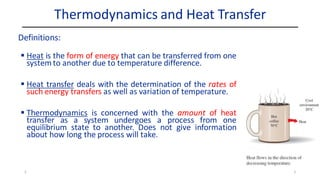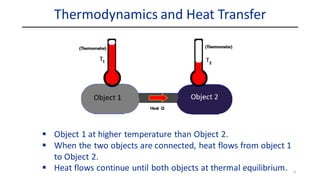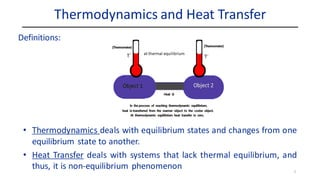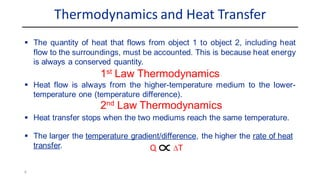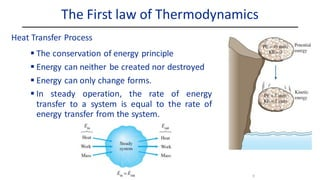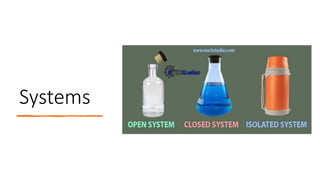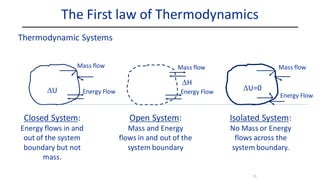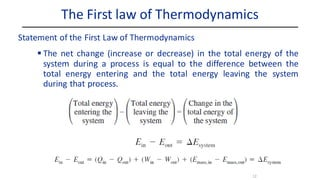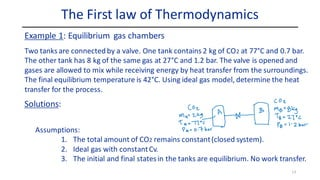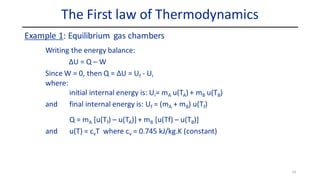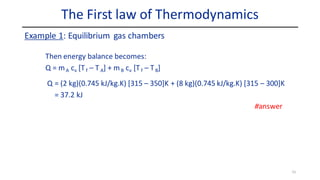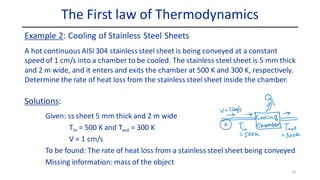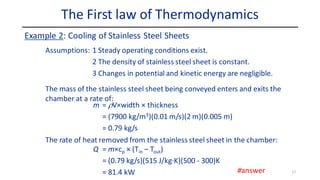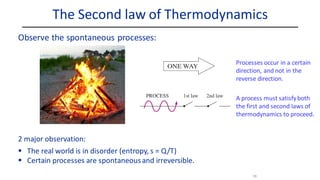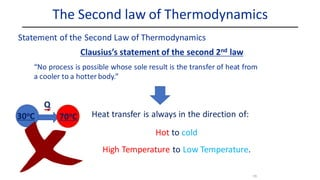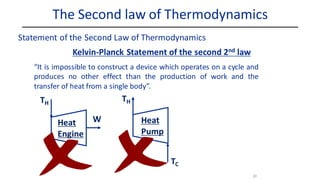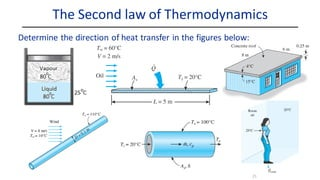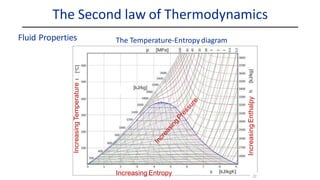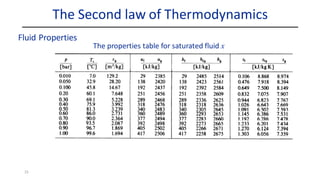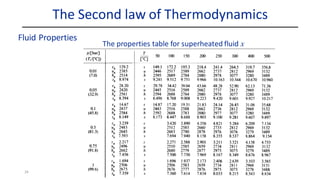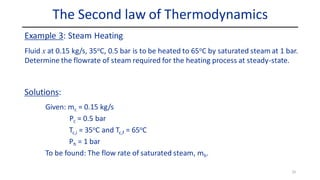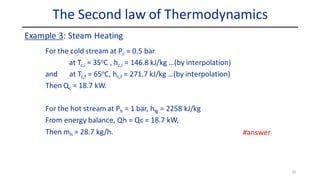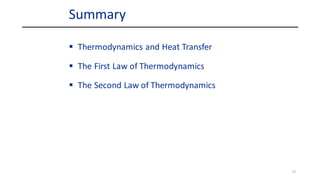1 von 27

### Principles-of-Thermodynamics

• 1. CH2043 Heat Transfer Processes and Equipment CC01, CC02, CC03, CC04 Wan Zaireen Nisa Yahya and Shafirah Samsuri 2022 English Program Ho Chi Minh City University of Technology
• 2. PRINCIPLES OF THERMODYNAMICS ▪ Thermodynamics and Heat Transfer ▪ The First Law of Thermodynamics ▪ The Second Law of Thermodynamics
• 3. Thermodynamics and Heat Transfer Stream Temperature Profiles 3 ▪ Heat Duties 60.1oC 150oC Heat Exchanger Duty, Q = 2529 kW T Streams need cooling Q Q = DH T Streams need heating Q Q = DH Hot Streams Cold Streams ▪ Stream profile on temperature (T) – heat (Q) diagram
• 4. 4 T Q Hot Streams Cold Streams DT1 DT2 Thermodynamics and Heat Transfer Stream profile for a heat exchanger 4
• 5. ▪ Heat is the form of energy that can be transferred from one systemto another due to temperature difference. ▪ Heat transfer deals with the determination of the rates of such energy transfers as well as variation of temperature. ▪ Thermodynamics is concerned with the amount of heat transfer as a system undergoes a process from one equilibrium state to another. Does not give information about how long the process will take. 5 Definitions: Thermodynamics and Heat Transfer 5
• 6. Thermodynamics and Heat Transfer ▪ Object 1 at higher temperature than Object 2. ▪ When the two objects are connected, heat flows from object 1 to Object 2. ▪ Heat flows continue until both objects at thermal equilibrium. 6
• 7. • Thermodynamics deals with equilibrium states and changes from one equilibrium state to another. • Heat Transfer deals with systems that lack thermal equilibrium, and thus, it is non-equilibrium phenomenon Thermodynamics and Heat Transfer Definitions: 7
• 8. ▪ The quantity of heat that flows from object 1 to object 2, including heat flow to the surroundings, must be accounted. This is because heat energy is always a conserved quantity. ▪ Heat flow is always from the higher-temperature medium to the lower- temperature one (temperature difference). ▪ Heat transfer stops when the two mediums reach the same temperature. ▪ The larger the temperature gradient/difference, the higher the rate of heat transfer. 8 2nd Law Thermodynamics Q DT 1st Law Thermodynamics Thermodynamics and Heat Transfer
• 9. 9 The First law of Thermodynamics ▪ The conservation of energy principle ▪ Energy can neither be created nor destroyed ▪ Energy can only change forms. ▪ In steady operation, the rate of energy transfer to a system is equal to the rate of energy transfer from the system. Heat Transfer Process
• 10. Systems
• 11. 11 The First law of Thermodynamics Thermodynamic Systems Closed System: Energy flows in and out of the system boundary but not mass. Mass flow Energy Flow DU Open System: Mass and Energy flows in and out of the system boundary Mass flow Energy Flow DH Isolated System: No Mass or Energy flows across the system boundary. Mass flow Energy Flow DU=0
• 12. 12 The First law of Thermodynamics ▪ The net change (increase or decrease) in the total energy of the system during a process is equal to the difference between the total energy entering and the total energy leaving the system during that process. Statement of the First Law of Thermodynamics
• 13. 13 Solutions: Two tanks are connected by a valve. One tank contains 2 kg of CO2 at 77°C and 0.7 bar. The other tank has 8 kg of the same gas at 27°C and 1.2 bar. The valve is opened and gases are allowed to mix while receiving energy by heat transfer from the surroundings. The final equilibrium temperature is 42°C. Using ideal gas model, determine the heat transfer for the process. Assumptions: 1. The total amount of CO2 remains constant(closed system). 2. Ideal gas with constantCv. 3. The initial and final statesin the tanks are equilibrium. No work transfer. Example 1: Equilibrium gas chambers The First law of Thermodynamics
• 14. 14 Writing the energy balance: ΔU = Q – W Since W = 0, then Q = ΔU = Uf - Ui where: initial internal energy is: Ui= mA u(TA) + mB u(TB) and final internal energy is: Uf = (mA + mB) u(Tf) Q = mA [u(Tf) – u(TA)] + mB [u(Tf) – u(TB)] and u(T) = cvT where cv = 0.745 kJ/kg.K (constant) Example 1: Equilibrium gas chambers The First law of Thermodynamics
• 15. 15 Then energy balance becomes: Q = mA cv [Tf – T A] + mB cv [Tf – TB] Q = (2 kg)(0.745 kJ/kg.K) [315 – 350]K + (8 kg)(0.745 kJ/kg.K) [315 – 300]K = 37.2 kJ Example 1: Equilibrium gas chambers The First law of Thermodynamics #answer
• 16. 16 Solutions: A hot continuous AISI 304 stainless steel sheet is being conveyed at a constant speed of 1 cm/s into a chamber to be cooled. The stainless steel sheet is 5 mm thick and 2 m wide, and it enters and exits the chamber at 500 K and 300 K, respectively. Determine the rate of heat loss from the stainless steel sheet inside the chamber. Given: ss sheet 5 mm thick and 2 m wide Tin = 500 K and Tout = 300 K V = 1 cm/s To be found: The rate of heat loss from a stainless steel sheet being conveyed Missing information: mass of the object Example 2: Cooling of Stainless Steel Sheets The First law of Thermodynamics
• 17. 17 Assumptions: 1 Steady operating conditions exist. 2 The density of stainless steel sheet is constant. 3 Changes in potential and kinetic energy are negligible. The mass of the stainless steel sheet being conveyed enters and exits the chamber at a rate of: Example 2: Cooling of Stainless Steel Sheets The First law of Thermodynamics m = rV×width × thickness = (7900 kg/m3)(0.01 m/s)(2 m)(0.005 m) = 0.79 kg/s The rate of heat removed from the stainless steel sheet in the chamber: Q = m×cp × (Tin – Tout) = (0.79 kg/s)(515 J/kg·K)(500 - 300)K = 81.4 kW #answer
• 18. 18 2 major observation: ▪ The real world is in disorder (entropy, s = Q/T) ▪ Certain processes are spontaneousand irreversible. The Second law of Thermodynamics Observe the spontaneous processes: Processes occur in a certain direction, and not in the reverse direction. A process must satisfyboth the first and second laws of thermodynamics to proceed.
• 19. 19 “No process is possible whose sole result is the transfer of heat from a cooler to a hotter body.” Clausius’s statement of the second 2nd law Heat transfer is always in the direction of: Hot to cold High Temperature to Low Temperature. The Second law of Thermodynamics Statement of the Second Law of Thermodynamics 30oC 70oC Q
• 20. 20 “It is impossible to construct a device which operates on a cycle and produces no other effect than the production of work and the transfer of heat from a single body”. Kelvin-Planck Statement of the second 2nd law The Second law of Thermodynamics Statement of the Second Law of Thermodynamics Heat Engine TH W Heat Pump TH TC
• 21. Determine the direction of heat transfer in the figures below: The Second law of Thermodynamics 21
• 22. Increasing Temperature Increasing Enthalpy The Temperature-Entropydiagram The Second law of Thermodynamics Fluid Properties 22 Increasing Entropy
• 23. 23 The properties table for saturated fluid x The Second law of Thermodynamics Fluid Properties
• 24. 24 The properties table for superheated fluid x The Second law of Thermodynamics Fluid Properties
• 25. 25 Solutions: Fluid x at 0.15 kg/s, 35oC, 0.5 bar is to be heated to 65oC by saturated steam at 1 bar. Determine the flowrate of steam required for the heating process at steady-state. Given: mc = 0.15 kg/s Pc = 0.5 bar Tc,i = 35oC and Tc,f = 65oC Ph = 1 bar To be found: The flow rate of saturated steam, mh. Example 3: Steam Heating The Second law of Thermodynamics
• 26. 26 For the cold stream at Pc = 0.5 bar at Tc,i = 35oC , hc,i = 146.8 kJ/kg …(by interpolation) and at Tc,f = 65oC, hc,f = 271.7 kJ/kg …(by interpolation) Then Qc = 18.7 kW. For the hot stream at Ph = 1 bar, hfg = 2258 kJ/kg From energy balance, Qh = Qc = 18.7 kW, Then mh = 28.7 kg/h. Example 3: Steam Heating The Second law of Thermodynamics #answer
• 27. Summary 27 ▪ Thermodynamics and Heat Transfer ▪ The First Law of Thermodynamics ▪ The Second Law of Thermodynamics